ISEE Lower Level Quantitative : How to compare fractions

Example Questions

← Previous 1 3 4 5 6

Example Question #11 : How To Order Fractions From Least To Greatest Or From Greatest To Least

Select the symbol to correctly fill in the blank below.__________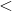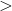Explanation:

To compare fractions, we need to first make common denominators.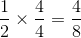Now that we have common denominators, we can compare numerators. The fraction with the bigger numerator has the greater value.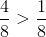Example Question #12 : How To Order Fractions From Least To Greatest Or From Greatest To Least

Select the symbol to correctly fill in the blank below.__________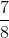Explanation:

To compare fractions, we need to first make common denominators.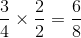Now that we have common denominators, we can compare numerators. The fraction with the bigger numerator has the greater value.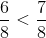Example Question #53 : Fractions

Select the symbol to correctly fill in the blank below.__________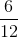Explanation:

To compare fractions, we need to first make common denominators.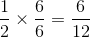Now that we have common denominators, we can compare numerators. The fraction with the bigger numerator has the greater value.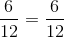Example Question #51 : Fractions

Select the symbol to correctly fill in the blank below.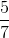__________Explanation:

To compare fractions, we need to first make common denominators.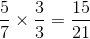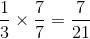Now that we have common denominators, we can compare numerators. The fraction with the bigger numerator has the greater value.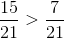Example Question #52 : Fractions

Select the symbol to correctly fill in the blank below.__________Explanation:

To compare fractions, we need to first make common denominators.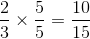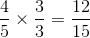Now that we have common denominators, we can compare numerators. The fraction with the bigger numerator has the greater value.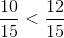Example Question #331 : Rational Numbers

Select the symbol to correctly fill in the blank below.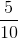__________Explanation:

To compare fractions, we need to first make common denominators.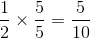Now that we have common denominators, we can compare numerators. The fraction with the bigger numerator has the greater value.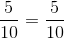Example Question #1 : Compare Two Fractions With Different Numerators And Different Denominators: Ccss.Math.Content.4.Nf.A.2

Select the symbol to correctly fill in the blank below.__________Explanation:

To compare fractions, we need to first make common denominators.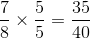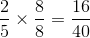Now that we have common denominators, we can compare numerators. The fraction with the bigger numerator has the greater value.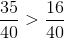Example Question #31 : Number & Operations: Fractions

Select the symbol to correctly fill in the blank below.__________Explanation:

To compare fractions, we need to first make common denominators.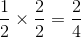Now that we have common denominators, we can compare numerators. The fraction with the bigger numerator has the greater value.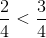Example Question #31 : Extend Understanding Of Fraction Equivalence And Ordering

Select the symbol to correctly fill in the blank below.__________Explanation:

To compare fractions, we need to first make common denominators.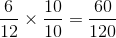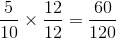Now that we have common denominators, we can compare numerators. The fraction with the bigger numerator has the greater value.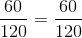Example Question #3 : Compare Two Fractions With Different Numerators And Different Denominators: Ccss.Math.Content.4.Nf.A.2

Select the symbol to correctly fill in the blank below.__________Explanation:

To compare fractions, we need to first make common denominators.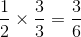Now that we have common denominators, we can compare numerators. The fraction with the bigger numerator has the greater value.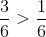← Previous 1 3 4 5 6

All ISEE Lower Level Quantitative Resources